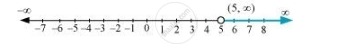CBSE (Arts) Class 11CBSE
Share

# Solve the Following Inequalities and Represent the Solution Graphically on Number Line: 3x – 7 > 2(X – 6), 6 – X > 11 – 2x - CBSE (Arts) Class 11 - Mathematics

ConceptGraphical Solution of Linear Inequalities in Two Variables

#### Question

Solve the following inequalities and represent the solution graphically on number line:

3x – 7 > 2(x – 6), 6 – x > 11 – 2x

#### Solution

3x – 7 > 2(x – 6)

⇒ 3x – 7 > 2x – 12

⇒ 3x – 2> – 12 + 7

⇒ x > –5 … (1)

6 – x > 11 – 2x

⇒ –x + 2x > 11 – 6

⇒ x > 5 … (2)

From (1) and (2), it can be concluded that the solution set for the given system of inequalities is (5, oo). The solution of the given system of inequalities can be represented on number line asIs there an error in this question or solution?

#### Video TutorialsVIEW ALL 

Solution Solve the Following Inequalities and Represent the Solution Graphically on Number Line: 3x – 7 > 2(X – 6), 6 – X > 11 – 2x Concept: Graphical Solution of Linear Inequalities in Two Variables.
S